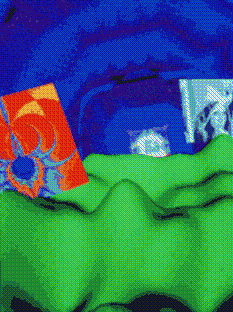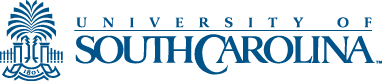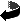MATH 599  Topics in Mathematics - Summer II, 2001 "Computational Science and Mathematics" Professor Sharpley Meets: M-F   8:30- 9:30 in Sumwalt 104/LeConte 313 Instructor Information  Office:   LeConte 313 D  Phone:   7-6268 Tuesday-Thursday - Continue on projects; random number generation. Friday - PowerWall Demo and Training (10 am)Course Information

Description:   The purpose of this course is to address the basic issues of computational science and mathematics. These issues include modeling physical processes through, for example, partial differential equations, the discretization of the PDE to provide numerical solutions, numerical analysis of the resulting computational algorithms, implementation on serial and parallel architectures, animated rendering of the time dependent solutions, and coupling to earlier procedures to refine the simulations. In addition to computational mathematics and the implementation of of parallel algorithms on IMI's Beowulf system, special topics will be introduced including computer graphics using openGL (for rendering of simulations on IMI's four processor ONYX 2), and virtual environments (using a number of tracking/steering devices) for the stereo PowerWall display.

Prerequisites:   Vector calculus; ordinary differential equations; experience in programming in C.

Reference Texts:

• "Solving Problems on Concurrent Processors," Vol. I,  G. Fox et al, Prentice Hall, Englewood Cliffs, NJ, 1988.
• "Parallel Programming with MPI", P.S. Pacheco, Morgan Kaufmann Publ,  San Francisco, CA, 1997.
• "Parallel and Distributed Computation, Numerical Methods", D.P. Bertsekas and J.N. Tsitsiklis, Prentice Hall, Englewood Cliffs, NJ, 1989.
• "Applied Mathematics, A Contemporary Approach", D. Logan, J. Wiley & Sons, New York, 1987.
• "Numerical Mathematics and Computing"(3-rd edition), W. Cheney and D. Kincaid, Brooks/Cole, Pacific Grove, CA, 1994.

Lectures:

• Lecture 1 (Tues. 7/10)
Computer architectures; von Neumann machines; pipelining, vector machines, Flynn's taxonomy: SISD (Monte Carlo apps.), SIMD, MIMD machines.

• Lecture 2 (Wed. 7/11)
Example SIMD pseudo code; communication topologies (ring, mesh, hypercube); communication switch models (crossbar, multistage, omega).

• Lecture 3 (Thur. 7/12)
Introduction to Numerical Analysis: proof of Taylors theorem with integral error term; solving
-uxx = f,  u(0)=u(1)=0
numerically by discretizing to get a tridiagonal linear system; Thomas' algorithm; developing a parallel version?

• Lecture 4 (Fri. 7/13)
Modeling a vibrating string: derivation of 2-nd order and one way wave equations; translations as solutions; discretization of the one way wave equation for a finite difference formulation; a numerical experiment to demonstrate instability and the need for further analysis.

• Lecture 5 (Mon. 7/16)
Numerical approximation of the 2-nd order wave equation

• Lecture 6 (Tues. 7/17)
Modeling heat flow: derivation of the multivariate heat equation; existence (Fourier series), uniqueness, continuity with respect to data.

• Lecture 7 (Wed. 7/18)
Modeling heat flow: (continued);

• Lecture 8 (Thur. 7/19)
Numerical approximation of the heat equation (explicit finite difference schemes); Experiments: error, stability, need for longer time step and higher accuracy.   Serial and Parallel implementations.

• Lecture 9 (Mon. 7/23)
MPI implementation of the heat equation (continued); Fourier series representations: computer experiments.

• Class Day 10 (Tues. 7/24)
MPI Lab projects: coding of sorting algorithm, Finite Difference Approximation to Heat Equation (explicit scheme), Matrix multiply.

• Lecture Day 11 (Wed. 7/25)
OpenGL, GLUT and Computer Graphics (Scott Johnson)

• Class 12 (Thur. 7/26)
Lab Day

• Class 13 (Fri. 7/27)
Update on MPI coding of algorithms; brief lecture on complex numbers.

• Lecture 14 (Mon. 7/30)
Properties of the continuous Fourier transform; the Discrete Fourier transform; signal filtering; Fourier inversion; interpolation with trig polynomials; a recursive algorithm for reducing the arithmetic complexity of computing the Fourier Transform.

• Class 15 (Tues. 7/31)
Continue on MPI Projects.

• Lecture 16 (Wed. 8/1)
Random number generation; serial and parallel algorithms; An Application. (John Lane)

• Lecture 17 (Thur. 8/2)
Random number generation and Monte Carlo applications (cont).

• Lecture 18 (Fri. 8/3)
FakeSpace Systems instructional sessions on haptic devices and operation of PowerWall.This page maintained by Robert Sharpley (sharpley@math.sc.edu) and last updated July 15, 2001. This page ©2000-2001, The Board of Trustees of the University of South Carolina. URL: http://www.math.sc.edu/~sharpley/math599

Return to Sharpley's Home Page